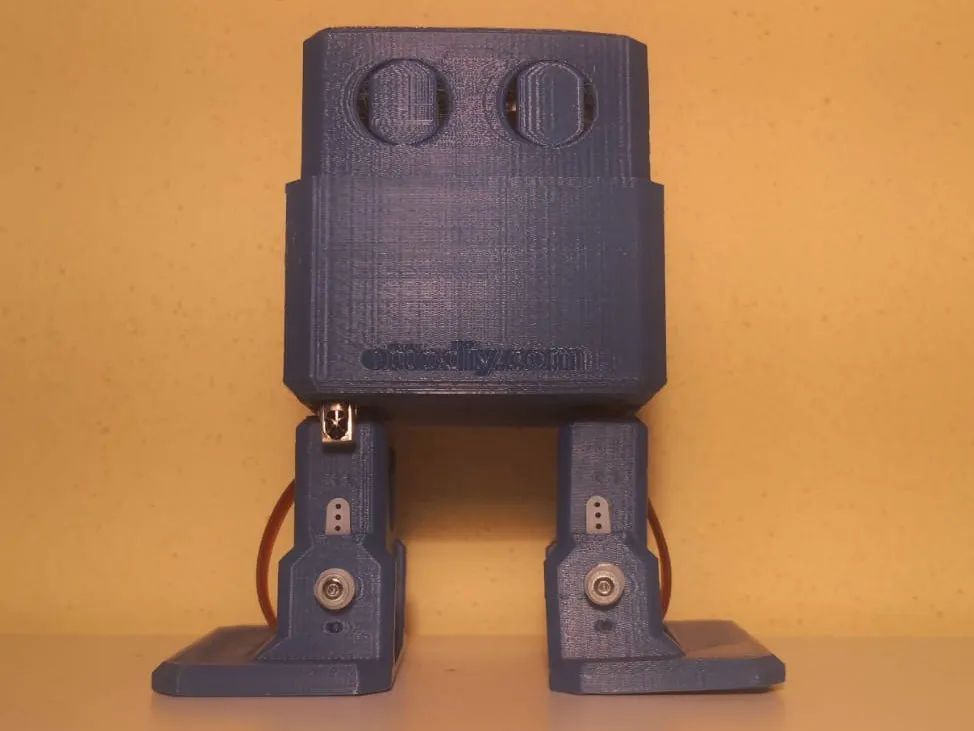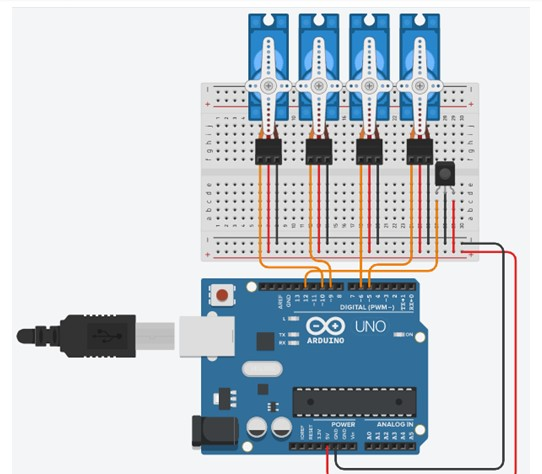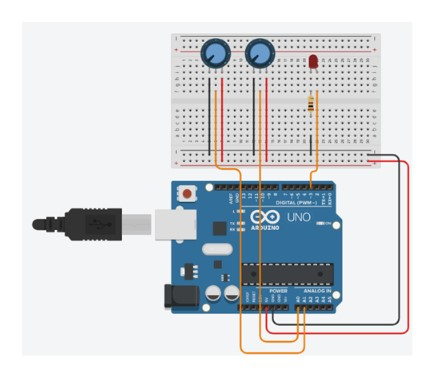Project tutorial# Otto Diy with Ir remote © GPL3+

Otto Diy is a bipedal robot, driven by microcontrollers and controlled by a remote, able to walk and turn around.

• 2,491 views
• 5 respects

## Necessary tools and machines3D Printer (generic)

## Apps and online services

### Introduction

In this report we will explain the operation of a bipedal robot driven by

microcontrollers and controlled by a remote, able to walk and turn around.

### Goal

The machines and projects must include the following features:

• use an Arduino microcontroller board (UNO, Leonardo, 101);

• request at least the use of an input sensor;

• generate at least one output interfacing with the real world, which can be visual (LED, screens) or physical (moving motors);

• use at least one device not explained in class, it can be a sensor or a motor and its pilot card.

The project we decided to build is a toy bipedal robot (taking the "Otto DIY" open source project as an example) able to walk and turn around using an Arduino board which is controlled by IR signals sent by another Arduino board (which will work as a remote control).

### Used Material

The components necessary for the realization of the project are the following ones:

• 2x Microcontroller (Arduino UNO, Elegoo Nano);
• 4x Micro servo SG90;
• IR LED;
• IR receiver VS1838B;
• joystick module;
• 100Ω resistance;
• Arduino nano expansion board;
• Jumper cables;
• PLA pieces (obtained by 3D printing).

The printed pieces’ 3D drawing files were downloaded from the website “Thingiverse”, as “Otto DIY” is an open source project.

The servos and the IR receiver don’t require any resistance as it’s integrated in the components.

To make the robot’s wirings easier we mounted the Elegoo Nano on an expansion board.

### Functioning

To make the bipedal robot we used 2 microcontrollers.

The first one is an Elegoo Nano board connected to the joystick module and to the IR LED to assume the function of a remote control: it calculates the position of the joystick to understand where the robot has to go (so, which code has to be sent).

The second microcontroller is the “mind” of the robot: it controls the movements of the 4 servos based on the infrared signals received by the sensor. The operation of both devices are described in the following flowcharts.

### Conclusion

To complete this project we had to deal with many problems and to learn how to solve them (for example, the Elegoo nano board didn’t have integrated connectors so, to make it work properly, we had to solder all the pins).

Other than that, we learned also how to work with components and technologies never studied before which was the main goal of the exercise.

## Code

##### Code RobotC#
This code is the Robot code, made on Arduino
```#include <IRremote.h>
#include <Otto9.h>

Otto9 Otto; //this is the name of our robot :)

IRrecv ir(12); //name and pin of the IR sensor
decode_results results; //this variable will assume the values of the recieved signals

#define PIN_LLEG 3 //YL - left leg pin
#define PIN_RLEG 4 //YR - right leg pin
#define PIN_LFOOT 2 //RL - left foot pin
#define PIN_RFOOT 5 //RR - right foot pin

#define PIN_Trigger  8 //US send pin
#define PIN_Echo     10 //US return pin
#define PIN_Buzzer  13 //buzzer pin

void setup() {

//initialisation of the robot by defining: YL, YR, RL, RR, load calibration (true or false), Noise sensor, Buzzer, Trigger, Echo
Otto.init(PIN_LLEG, PIN_RLEG, PIN_LFOOT, PIN_RFOOT, false, A6, PIN_Buzzer, PIN_Trigger, PIN_Echo);
Otto.home(); //set all the servos to 90

delay(500);
Serial.begin(9600);
ir.enableIRIn(); //start to read the IR sensor values
}

void loop() {

if (ir.decode(&results)) {

if (results.value == 24) { //if the code sent by the remote is 24:
Otto.walk(8, 600, 1); //walk: 8=steps, 600=period (time per step), 1=direction (forward)
Serial.println("forward");
}

if (results.value == 32) { //if the code sent by the remote is 32:
Otto.walk(8, 600, -1); //walk: 8=steps, 600=period (time per step), -1=direction (backwards)
Serial.println("backwards");
}
if (results.value == 8) { //if the code sent by the remote is 8:
Otto.turn(8, 800, 1); //turn: 8=steps, 600=period (time per step), -1=direction (right)
Serial.println("right");
}
if (results.value == 16) { //if the code sent by the remote is 16:
Otto.turn(8, 800, -1); //turn: 8=steps, 600=period (time per step), -1=direction (left)
Serial.println("left");
}
ir.resume(); //start reading the sensor values again
}
else { //if the robot doesn't recieve any signal
Otto.home();
}
results.value = 0;
}
```
##### Code RemoteC#
This code is the Remote code, used for driving the Robot, made on Arduino.
```#include<IRremote.h>

IRsend emitter; //this is the name of the IR LED

//the following variables are necessary for the remote to understand the position of the joystick
int x = 0;
int y = 0;
int xPos = 0; //right side of the X axis
int xNeg = 0; //left side of the X axis
int yPos = 0; //right side of the Y axis
int yNeg = 0; //left side of the Y axis

void setup()
{
Serial.begin(9600);
}

void loop()
{

//read the joystick's X axis & Y axis values

//these are the operations to calculate Xpos & Xneg
if (x > 524) {
xPos = (x - 512) / 2;
xNeg = 0;
}
else if (x < 500) {
xPos = 0;
xNeg = (511 - x) / 2 ;
}
else {
xPos = 0;
xNeg = 0;
}

//these are the operations to calculate Ypos & Yneg
if (y > 524) {
yPos = (y - 512) / 2;
yNeg = 0;
}
else if (y < 500) {
yPos = 0;
yNeg = (511 - y) / 2;
}
else {
yPos = 0;
yNeg = 0;
}

//tell otto to turn right
if ((xPos - xNeg - yPos - yNeg) > 0) {
emitter.sendSony(0x8, 32);
Serial.println("right");
delay(40);
//tell otto to turn left
}
if ((xNeg - yPos - yNeg - xPos) > 0) {
emitter.sendSony(0x10, 32);
Serial.println("left");
delay(40);
}
//tell otto to walk forward
if ((yPos - yNeg - xPos - xNeg) > 0) {
emitter.sendSony(0x18, 32);
Serial.println("forward");
delay(40);
//tell otto to walk backwards
}
if ((yNeg - xPos - xNeg - yPos) > 0) {
emitter.sendSony(0x20, 32);
Serial.println("backwards");
delay(40);
}

}
```

## Custom parts and enclosures

##### 3D Printed parts
The parts we used to assembly the robot.

## Schematics

This is the robot circuit, made on Tinkercadthis is the Remote circuit, made on TinkercadFebruary 9, 2020

#### Members who respect this project

See similar projects
you might like

#### DIY IR Remote and IR Remote controlled LEDs

Project tutorial by Rucksikaa Raajkumar

• 3,196 views
• 7 respects

• 16,701 views
• 1 comment
• 26 respects

#### Otto DIY Humanoid Robot (alike)

Project tutorial by Camilo Parra Palacio

• 7,522 views
• 22 respects

• 4,192 views
• 6 respects

#### Otto DIY build your own robot in one hour!

Project tutorial by Camilo Parra Palacio

• 193,742 views